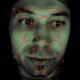# Recursive 2D Structure

Actionscript:
1. [SWF(width = 600, height = 700, frameRate=24)]
2. var canvas:BitmapData = new BitmapData(stage.stageWidth,stage.stageHeight,false, 0xFFFFFF);
4.
5. var maxBranches:int = 600;
6. var branches:int = 0;
7. var startX:Number = 300
8. makeBranch(startX,690,30,-60, 60);
9.
10. function makeBranch(xp:Number, yp:Number, step:Number, min:Number, max:Number):void {
11.     var vectors:Shape = Shape(addChild(new Shape()));
12.     var cX:Number, cY:Number, eX:Number, eY:Number
13.     var dcX:Number=xp, dcY:Number=yp, deX:Number=xp, deY:Number=yp;
14.     var theta:Number = (min + Math.random()*(max-min) - 90) * Math.PI / 180;
15.     cX = xp + step * Math.cos(theta);
16.     cY = yp + step * Math.sin(theta);
17.     theta = (min + Math.random()*(max-min)-90) * Math.PI / 180;
18.     eX = cX + step * Math.cos(theta);
19.     eY = cY + step * Math.sin(theta);
20.     var run:Function = function():void{
21.          dcX +=  (cX - dcX) / 2;
22.          dcY +=  (cY - dcY) / 2;
23.          deX +=  (eX - deX) / 8;
24.          deY +=  (eY - deY) / 8;
25.          with(vectors.graphics){
26.               clear();
27.               beginFill(0xFFFFFF,0.8);
28.               lineStyle(0,0x000000,0.8);
29.               moveTo(startX, yp);
30.               lineTo(xp, yp);
31.               curveTo(dcX, dcY, deX, deY);
32.               lineTo(startX, deY);
33.          }
34.          if (Math.abs(dcX - cX) <1 && Math.abs(deX - eX) <1 && Math.abs(dcY - cY) <1 && Math.abs(deY - eY) <1){
35.              canvas.draw(vectors);
36.              removeChild(vectors);
37.              if (branches <maxBranches){
38.                  setTimeout(makeBranch, 10, deX, deY, step - Math.random(), -90, 90);
39.                  branches++;
40.                  if (int(Math.random()*2) == 1){
41.                     setTimeout(makeBranch, 10, deX, deY, step - Math.random()*3, -90, 90);
42.                     branches++;
43.                  }
44.              }
45.          }else{
46.              setTimeout(arguments.callee,  1000 / 24);
47.          }
48.     }();
49. }

This snippet uses a technique similar to what you might use to create a recursive tree. A bit of additional logic is added for bezier branches, filled shapes and animation.

WARNING: may run slow on older machines

Have a look at the swf...1.Mohammad Haseeb
Posted July 21, 2009 at 10:52 am | Permalink

Good work there as always Zevan. You might wanna put a warning on snippets with high processor usage. This one uses recursion and can cause the browser or the system to hang.

2.Zevan
Posted July 21, 2009 at 11:09 am | Permalink

Thanks Mohammad. I’ll add a little warning - did it hang on your machine? On my macbook pro 2.4 ghz the flash player goes to 50% cpu usage…

3.Mario Gonzalez
Posted July 21, 2009 at 1:28 pm | Permalink

It did on mine at home, if you don’t have dual core, 50% equals 100% lol

4.Og2t
Posted July 22, 2009 at 12:57 am | Permalink

This is beautiful Zevan! Went quite fast on mine iMac 2Ghz.

5.Zevan
Posted July 22, 2009 at 3:35 am | Permalink

thanks Og2t

6.Mohammad Haseeb
Posted July 22, 2009 at 9:28 am | Permalink

I have a Corei7 920 Processor and a super-clocked GTX 260 running on my machine with Windows 7. I tried running this using IE, Firefox and Safari (on my hackmac) and all of the browsers hung. 50% of the processor was dedicated to the process. IE 8 was the only browser that stopped the script after giving me a warning.

I’d suggest two things:
- Put a limit on the recursion.
- Put Idle cycles in the loop, which would allow the processor to cool down.

7.Zevan
Posted July 22, 2009 at 9:38 am | Permalink

That’s odd… just tested it on my old 1.2 ghz windows machine and it ran slow, but it didn’t hang. What version of the flash player are you using?

There is a limit on the recursion and it can be tweaked in the code by changing the maxBranches. The idle cycles aren’t necessary as you can just change the delay on line 41 from 10 ms to 100ms… or you could even use a slightly random ms so that not all the branches are running at the same time.

Anyone else experience a hang like the one Mohammad describes?